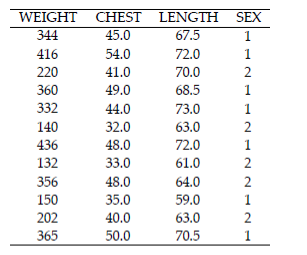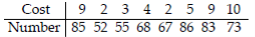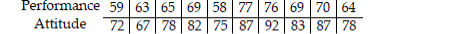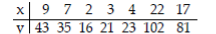• FAQ
• Contact/
/
/
Given the linear correlation coefficient r and the sample size
Not my Question
Flag Content

# Question : Given the linear correlation coefficient r and the sample size : 1728910

Given the linear correlation coefficient r and the sample size n, determine the critical values of r and use your finding to state whether or not the given r represents a significant linear correlation. Use a significance level of 0.05.

1) r = 0.543, n = 25

A) Critical values: r = ±0.487 , no significant linear correlation

B) Critical values: r = ±0.396 , no significant linear correlation

C) Critical values: r = ±0.487 , significant linear correlation

D) Critical values: r = ±0.396 , significant linear correlation

Use the given information to find the coefficient of determination.

2) A regression equation is obtained for a collection of paired data. It is found that the total variation is 20.711, the explained variation is 18.592, and the unexplained variation is 2.119. Find the coefficient of determination.

A) 1.114 B) 0.102 C) 0.898 D) 0.114

Find the value of the linear correlation coefficient r.

3) The paired data below consist of the temperatures on randomly chosen days and the amount a certain kind of plant grew (in millimeters):

Temp 62 76 50 51 71 46 51 44 79

Growth 36 39 50 13 33 33 17 6 16

A) 0 B) 0.196 C) -0.210 D) 0.256

Use computer software to find the multiple regression equation. Can the equation be used for prediction?

4) A wildlife analyst gathered the data in the table to develop an equation to predict the weights of

bears. He used WEIGHT as the dependent variable and CHEST, LENGTH, and SEX as the independent variables. For SEX, he used male=1 and female=2.A) WEIGHT = 196 + 2.35CHEST + 3.40LENGTH + 25SEX; Yes, because the R2 is high

B) WEIGHT = -320 + 10.6CHEST + 7.3LENGTH - 10.7SEX; Yes, because the P-value is high

C) WEIGHT = -442.6 + 12.1CHEST + 3.6LENGTH - 23.8SEX; Yes, because the adjusted R2 is high

D) WEIGHT = 442.6 + 12.1CHEST + 4.2LENGTH - 21SEX; Yes, because the P-value is low

Solve the problem.

5) A regression equation can be used to make predictions of the y value corresponding to a particular x value. Determine whether the following statement is true or false:

The 95% confidence interval for the mean of all values of y for which x = x0 will be wider than the 95% confidence interval for a single y for which x = x0.

A) True B) False

Find the value of the linear correlation coefficient r.

6) The paired data below consist of the costs of advertising (in thousands of dollars) and the number of products sold (in thousands):A) 0.708 B) 0.246 C) -0.071 D) 0.235

7) Managers rate employees according to job performance and attitude. The results for several randomly selected employees are given below.A) 0.729 B) 0.916 C) 0.863 D) 0.610

Provide an appropriate response.

8) A regression equation is obtained for a set of data. After examining a scatter diagram, the researcher notices a data point that is potentially an influential point. How could she confirm that this data point is indeed an influential point?

Find the explained variation for the paired data.

9) The equation of the regression line for the paired data below is y = 6.18286 + 4.33937x. Find the 9) explained variation.A) 13.479 B) 6,531.37 C) 6,544.86 D) 6,421.83

## Solution 5 (1 Ratings )

Solved
Statistics 10 Months Ago 36 Views